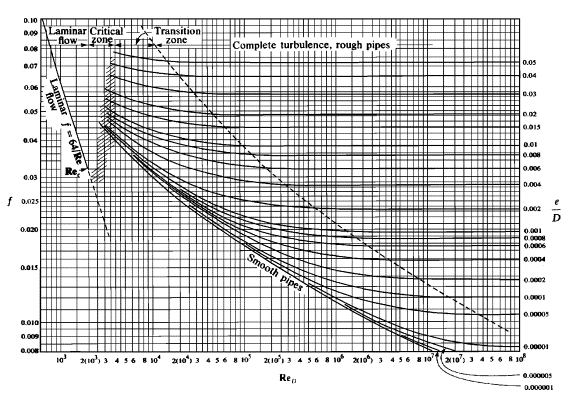# Fluid Mechanics - Estimating Reynolds number and flow rate.

• jc213

## Homework Statement

A pipe 0.15m in diameter has a roughness ratio (k/d) of 0.002 and carries water at a flow rate Q.

Using the moody diagram, show that at high flow rates (where the friction factor is independent of reynolds number), the head loss per m length due to friction is approx 25.7(Q^2).

Estimate the lowest value of reynolds number and hence Q for which this result is valid.

## The Attempt at a Solution

I have already proved the first part of the question using the darcy-weisbach formula. But now I am unsure how to use that result in order to estimate the lowest value of reynolds number and flow rate. I know it involves reading from the moody diagram and I'm fairly sure it will be an iterative process, but I am stuck on how to actually go about it.

Any help would be really appreciated.

Thanks

EDIT:

If it helps the answers I have been given are

Re~ 6 x 10^5
Q~ 0.0807

Last edited:
Hi jc, welcome to the board.
Actually, it's not iterative. You can determine On a Moody diagram like this one:There's a zone on the right where it says "complete turbulence" and the lines are roughly horizontal. Where the dotted line separates the completely turbulent zone from the transition zone is where the increase in Re no longer changes the friction factor.